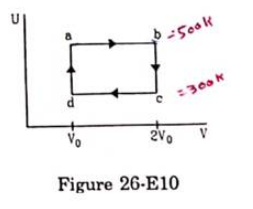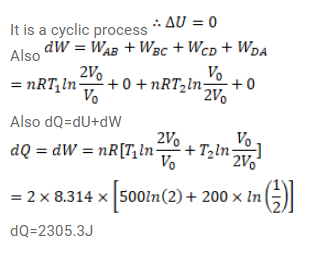# Figure (26-E10) shows the variation in the internal energy UQuestion:

Figure (26-E10) shows the variation in the internal energy $U$ with the volume $\mathrm{V}$ of $2.0$ mole of an ideal gas in a cyclic process abcda. The temperature of the gas at $b$ and c are $500 \mathrm{k}$ and $300 \mathrm{k}$ respectively, Calculate the heat absorbed by the gas during the process.Solution: